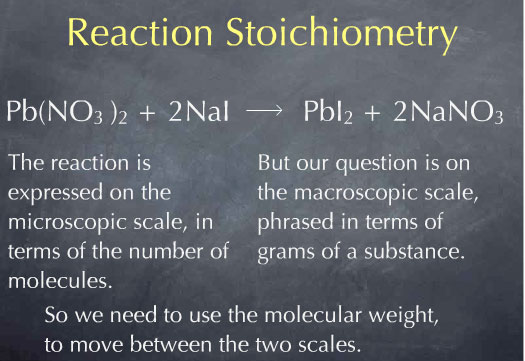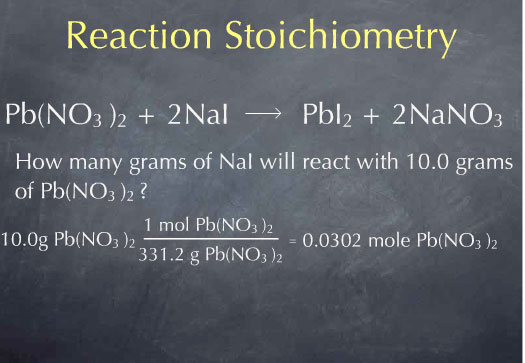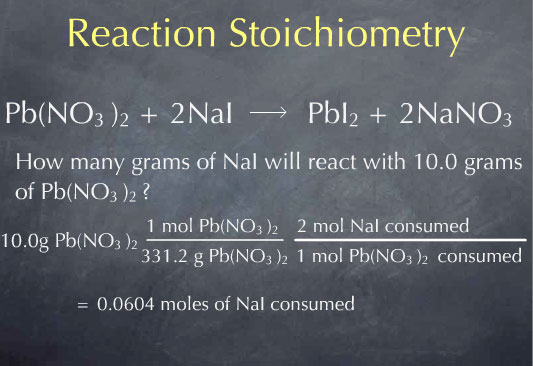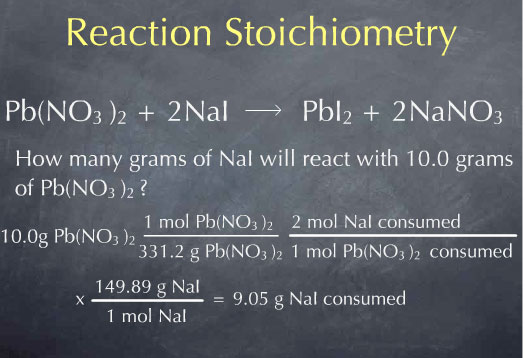# Stoichiometry Tutorials: Reaction Stoichiometry

(from a complete OLI stoichiometry course)

Reaction stoichiometry allows us to determine the amount of substance that is consumed or produced by a reaction. The following video considers the first part of this: how much of a reactant is consumed in a chemical reaction. Product formation is discussed elsewhere.

### Reaction Stochiometry Movie Text

Stoichiometry was first discovered by Jeremias Richter, a German chemist. It was Richter who coined the term stoichiometry, a tongue-twisting word that baffles students to this day. Stoichiometry was derived from stoikheion, Greek for "element", and "metron", meaning measure.

What Richter discovered was that it was possible to quantify the amount of substances consumed or produced by a chemical reaction. Richter was working with the following reaction of magnesium oxide neutralizing sulfuric acid. He figured out that there was a definite proportion between the amount of magnesium oxide and the amount of sulfuric acid with which it reacted. He phrased his conclusion as: "615 parts by weight of Magnesium Oxide were needed to neutralize 1000 parts by weight of Sulfuric Acid."

In other words, If I had 1000 grams of sulfuric acid - a kilogram - it would take 615 grams of magnesium oxide to neutralize it. If I had 2000 grams of sulfuric acid, I would have to use twice as much magnesium oxide, or 1230 grams of magnesium oxide to neutralize it.

So how do we think about this in modern terms? We now know that magnesium oxide is a molecule consisting of one magnesium atom and one oxygen atom, MgO, and that sulfuric acid is the molecule H2SO4. We also know that the neutralization reaction produces MgSO4 and water.

MgO + H2SO4 → MgSO4 + H2O

We can now look at this from a molecular perspective and say that one molecule of magnesium oxide reacts with one molecule of sulfuric acid, so there is a one to one reaction between these molecules.

One molecule MgO : One molecule H2SO4

Richter's discovery of specific proportions is a consequence of this one to one reaction.

Before moving forward, let's switch to a different reaction that is not one to one:

Pb(NO3)2 + 2 NaI → PbI2 + 2 NaNO3

Consider the following question: how many grams of NaI will react with 10.0 g of Pb(NO3)2? This is the type of question that reaction stoichiometry can help us answer.Let me go back to our original question: how many grams of NaI will react with 10.0 g of Pb(NO3)2? I start with my given quantity: 10.0 g of Pb(NO3)2. Our question is on the macroscopic scale: it is phrased in terms of grams of substance. However, the reaction, which tells us that one molecule of Pb(NO3)2 reacts with two molecules of NaI, is expressed on the microscopic scale: it is phrased in terms of numbers of molecules. The molecular weight will be very useful here, since it allows us to go back and forth between the mass of a substance and the number of molecules of that substance.Multiplying by 1 mole of Pb(NO3)2 for every 331.2 grams of Pb(NO3)2, I obtain 0.0311 moles of Pb(NO3)2. I now know the number of molecules of Pb(NO3)2 present in 10.0 grams of Pb(NO3)2. Now that Iâ€™m at the molecular scale, I can use the chemical reaction to determine how many molecules of NaI will react with this many molecules of Pb(NO3)2. The chemical reaction tells me that 2 molecules of NaI are consumed for every molecule of Pb(NO3)2 that is consumed. This is captured by the ratio: 2 moles of NaI consumed for every 1 mole of Pb(NO3)2 consumedThis gives me the amount of NaI consumed on the molecular scale: 0.0604 moles. To get to the macroscopic scale, I again use the molecular weight:Multiplying by 149.89 grams of NaI per mole of NaI, I find that 0.905 grams of NaI are consumed.

I've used the chemical reaction, which tells me that at the molecular scale 2 mol of NaI are consumed for every 1 mol of Pb(NO3)2 consumed. I converted to this to the macroscopic scale to find that 9.05 g of NaI are consumed for every 10.0 g of Pb(NO3)2 consumed. This type of macroscopic relationship is the type of macroscopic relation that Richter discovered back in the 1790's. Richter was the first person to discover and quantify this type of relationship, and he laid out the basic principles of stoichiometry that we use to this day. This was long before the molecular structure of matter was understood, so his results were all phrased at the macroscopic scale of grams.

Unfortunately, Richter was a bad writer and his papers were hard to read. It took about 5 years before people began to really read his work and be influenced by it. Once it began to be read, it did not take long for some scientists to realize that Richter's definite proportions could be explained by assuming that molecules exist. They hypothesized that matter consists of molecules and that these molecules react though chemical equations with simple coefficients, such as those seen here in which two molecules of NaI react with one molecule of Pb(NO3)2.

However, it took another 100 years to fully develop the molecular theory of matter to a point where it was accepted by most scientists. Richter's discover of stoichiometry was an important first step along the way to developing the modern molecular view of chemistry.